# RD Sharma Solutions for Class 11 Chapter 19 - Arithmetic Progressions Exercise 19.1

This exercise mainly deals with problems based on arithmetic progression (A.P.). To make learning Maths fun experts have explained the topics in easily understandable steps. For students to understand these concepts, solved examples are available before the exercise wise problems. The solutions pdf mainly contain answers with explanations in an interactive manner to help students perform well in the board exam. To get a clear idea about the method of solving problems, students can use RD Sharma Class 11 Maths Solutions pdf, from the links given below.

## Download the pdf of RD Sharma Solutions for Class 11 Maths Exercise 19.1 Chapter 19 – Arithmetic Progressions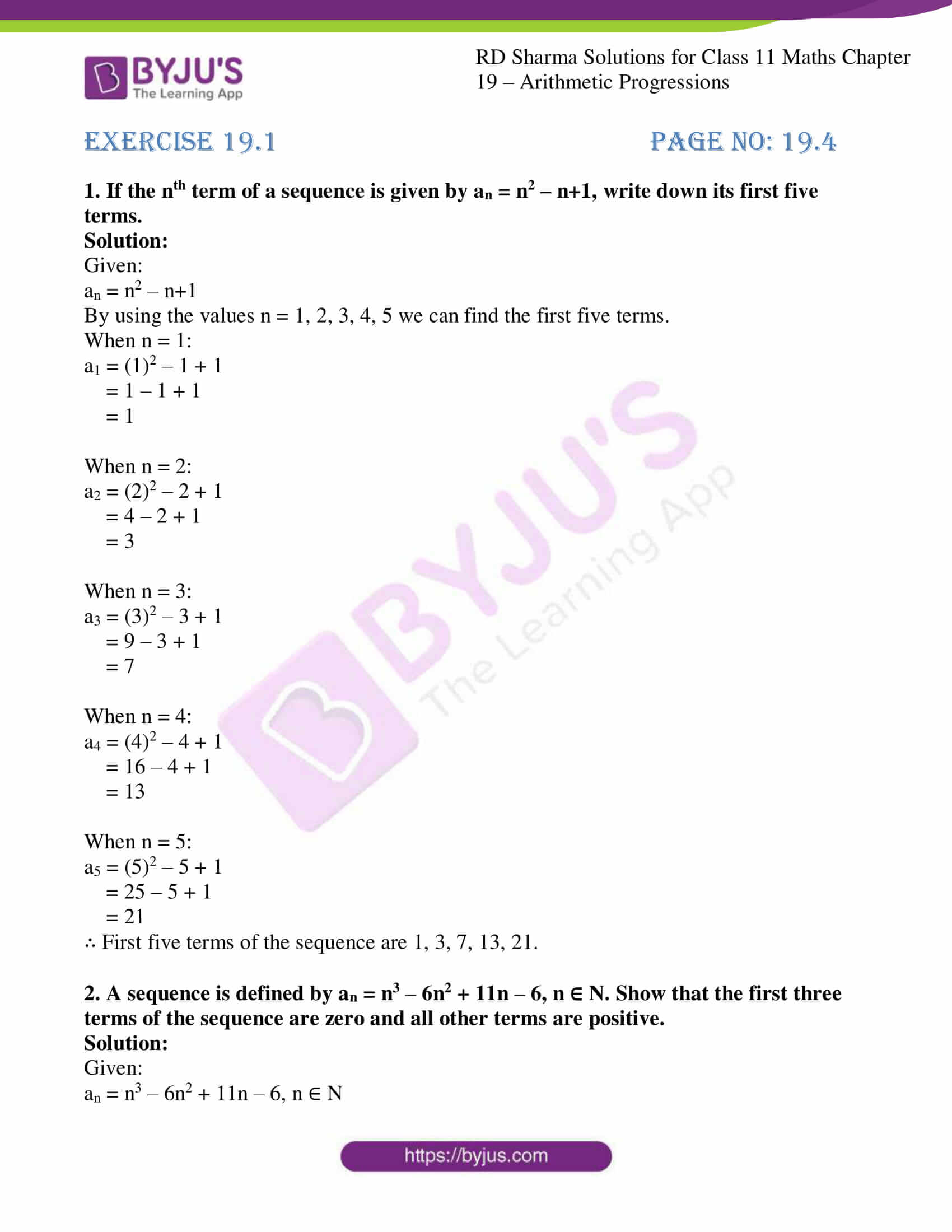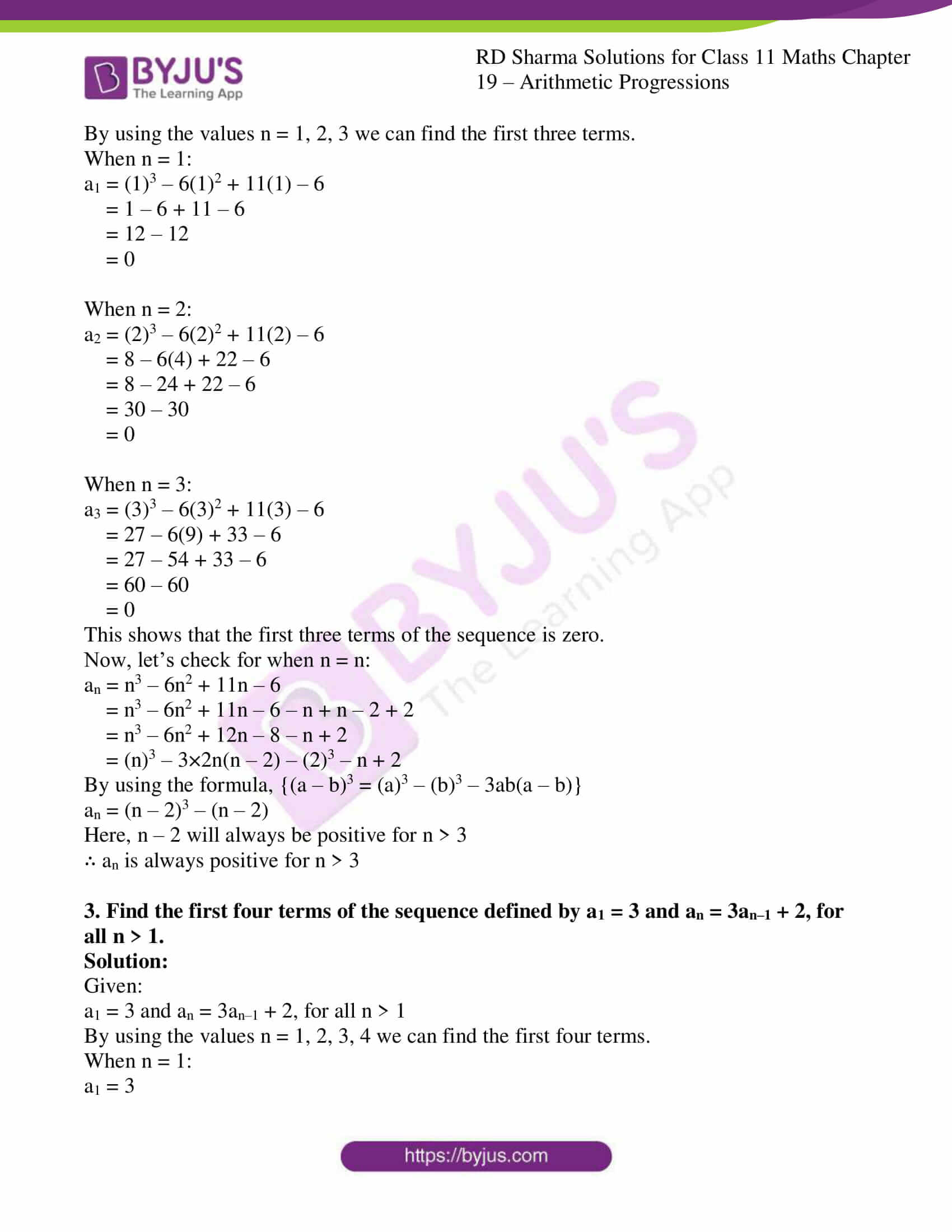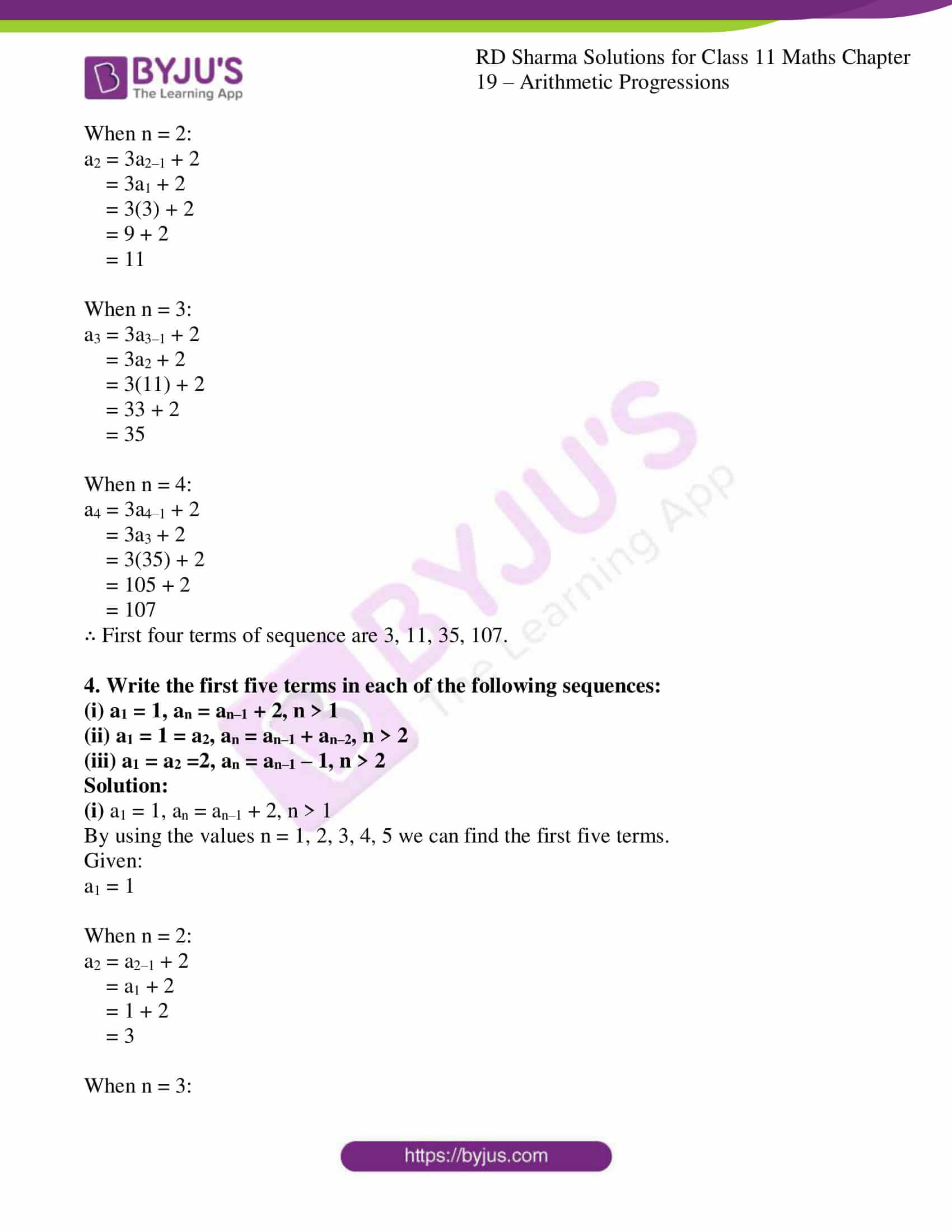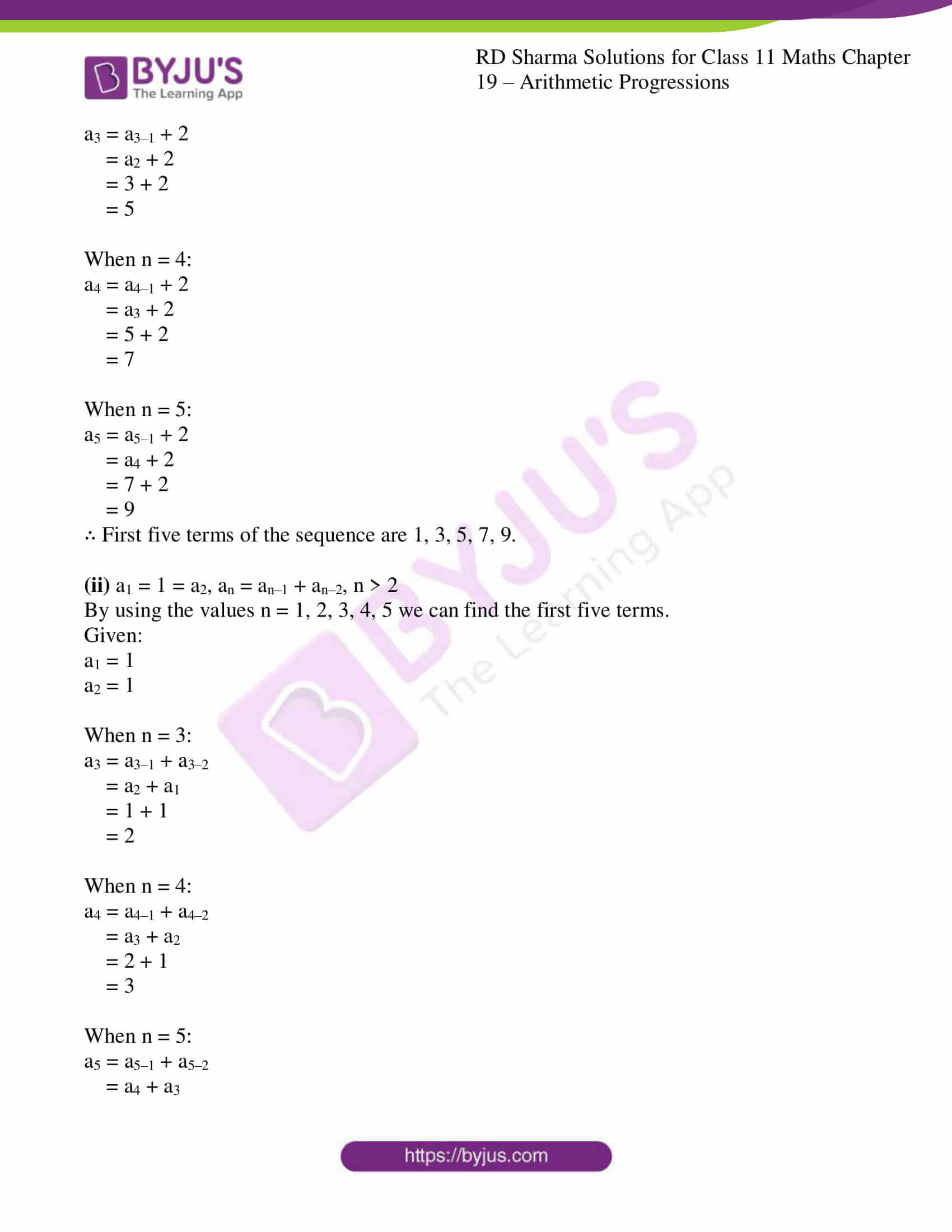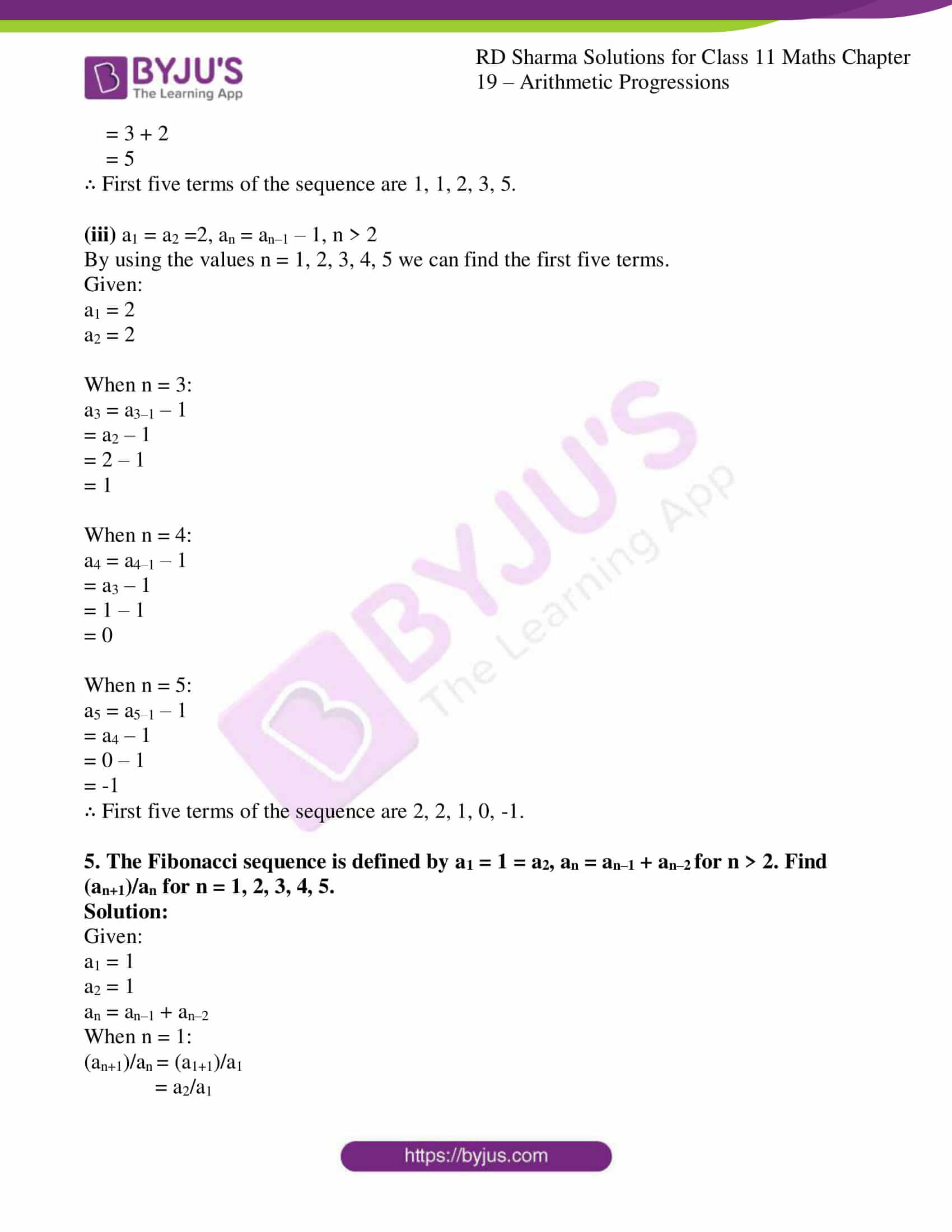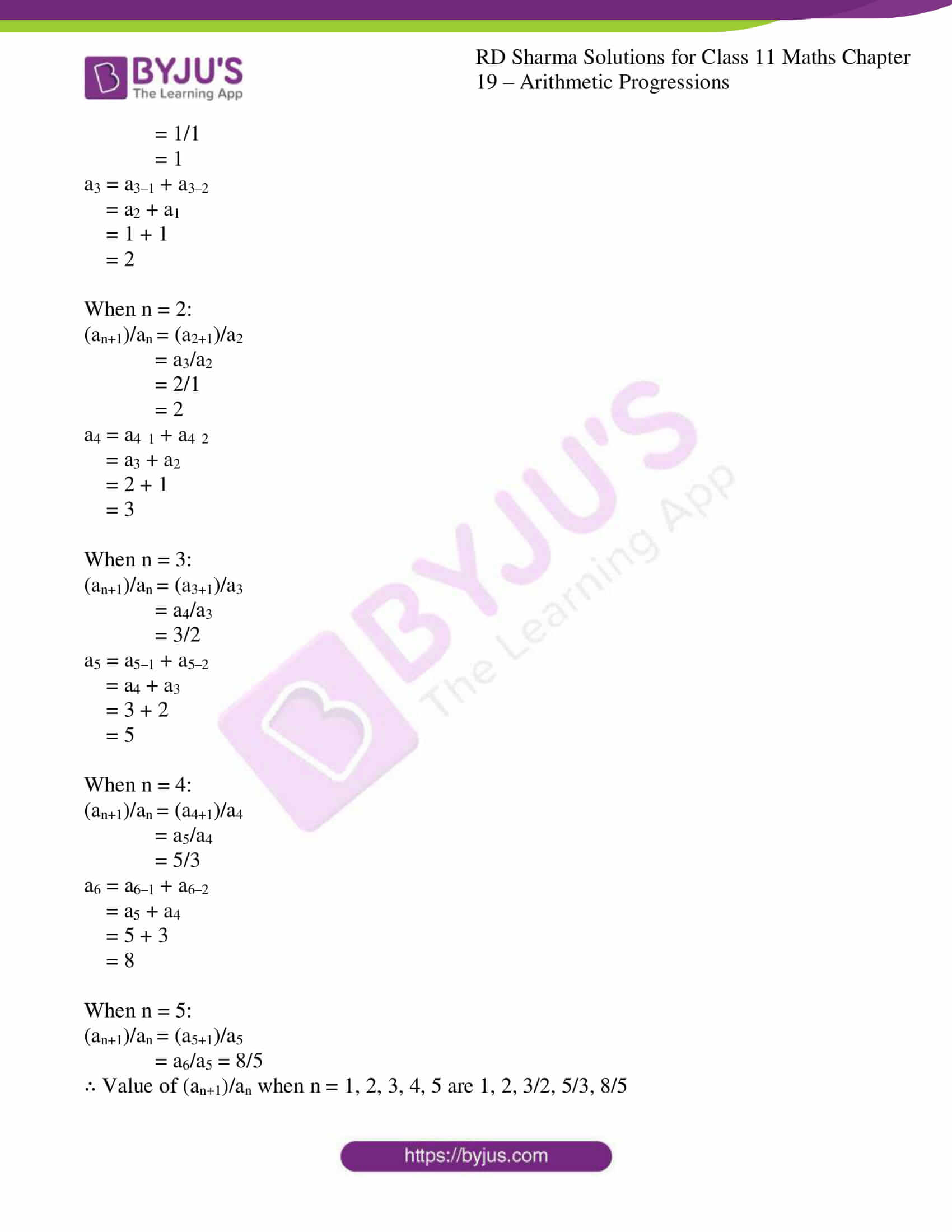### Access answers to RD Sharma Solutions for Class 11 Maths Exercise 19.1 Chapter 19 – Arithmetic Progressions

1. If the nth term of a sequence is given by an = n2 – n+1, write down its first five terms.

Solution:

Given:

an = n2 – n+1

By using the values n = 1, 2, 3, 4, 5 we can find the first five terms.

When n = 1:

a1 = (1)2 – 1 + 1

= 1 – 1 + 1

= 1

When n = 2:

a2 = (2)2 – 2 + 1

= 4 – 2 + 1

= 3

When n = 3:

a3 = (3)2 – 3 + 1

= 9 – 3 + 1

= 7

When n = 4:

a4 = (4)2 – 4 + 1

= 16 – 4 + 1

= 13

When n = 5:

a5 = (5)2 – 5 + 1

= 25 – 5 + 1

= 21

∴ First five terms of the sequence are 1, 3, 7, 13, 21.

2. A sequence is defined by an = n3 – 6n2 + 11n – 6, n ∈ N. Show that the first three terms of the sequence are zero and all other terms are positive.

Solution:

Given:

an = n3 – 6n2 + 11n – 6, n ∈ N

By using the values n = 1, 2, 3 we can find the first three terms.

When n = 1:

a1 = (1)3 – 6(1)2 + 11(1) – 6

= 1 – 6 + 11 – 6

= 12 – 12

= 0

When n = 2:

a2 = (2)3 – 6(2)2 + 11(2) – 6

= 8 – 6(4) + 22 – 6

= 8 – 24 + 22 – 6

= 30 – 30

= 0

When n = 3:

a3 = (3)3 – 6(3)2 + 11(3) – 6

= 27 – 6(9) + 33 – 6

= 27 – 54 + 33 – 6

= 60 – 60

= 0

This shows that the first three terms of the sequence is zero.

Now, let’s check for when n = n:

an = n3 – 6n2 + 11n – 6

= n3 – 6n2 + 11n – 6 – n + n – 2 + 2

= n3 – 6n2 + 12n – 8 – n + 2

= (n)3 – 3×2n(n – 2) – (2)3 – n + 2

By using the formula, {(a – b)3 = (a)3 – (b)3 – 3ab(a – b)}

an = (n – 2)3 – (n – 2)

Here, n – 2 will always be positive for n > 3

∴ an is always positive for n > 3

3. Find the first four terms of the sequence defined by a1 = 3 and an = 3an–1 + 2, for all n > 1.

Solution:

Given:

a1 = 3 and an = 3an–1 + 2, for all n > 1

By using the values n = 1, 2, 3, 4 we can find the first four terms.

When n = 1:

a1 = 3

When n = 2:

a2 = 3a2–1 + 2

= 3a1 + 2

= 3(3) + 2

= 9 + 2

= 11

When n = 3:

a3 = 3a3–1 + 2

= 3a2 + 2

= 3(11) + 2

= 33 + 2

= 35

When n = 4:

a4 = 3a4–1 + 2

= 3a3 + 2

= 3(35) + 2

= 105 + 2

= 107

∴ First four terms of sequence are 3, 11, 35, 107.

4. Write the first five terms in each of the following sequences:
(i) a1 = 1, an = an–1 + 2, n > 1

(ii) a1 = 1 = a2, an = an–1 + an–2, n > 2

(iii) a1 = a2 =2, an = an–1 – 1, n > 2

Solution:

(i) a1 = 1, an = an–1 + 2, n > 1

By using the values n = 1, 2, 3, 4, 5 we can find the first five terms.

Given:

a1 = 1

When n = 2:

a2 = a2–1 + 2

= a1 + 2

= 1 + 2

= 3

When n = 3:

a3 = a3–1 + 2

= a2 + 2

= 3 + 2

= 5

When n = 4:

a4 = a4–1 + 2

= a3 + 2

= 5 + 2

= 7

When n = 5:

a5 = a5–1 + 2

= a4 + 2

= 7 + 2

= 9

∴ First five terms of the sequence are 1, 3, 5, 7, 9.

(ii) a1 = 1 = a2, an = an–1 + an–2, n > 2

By using the values n = 1, 2, 3, 4, 5 we can find the first five terms.

Given:

a1 = 1

a2 = 1

When n = 3:

a3 = a3–1 + a3–2

= a2 + a1

= 1 + 1

= 2

When n = 4:

a4 = a4–1 + a4–2

= a3 + a2

= 2 + 1

= 3

When n = 5:

a5 = a5–1 + a5–2

= a4 + a3

= 3 + 2

= 5

∴ First five terms of the sequence are 1, 1, 2, 3, 5.

(iii) a1 = a2 =2, an = an–1 – 1, n > 2

By using the values n = 1, 2, 3, 4, 5 we can find the first five terms.

Given:

a1 = 2

a2 = 2

When n = 3:

a3 = a3–1 – 1

= a2 – 1

= 2 – 1

= 1

When n = 4:

a4 = a4–1 – 1

= a3 – 1

= 1 – 1

= 0

When n = 5:

a5 = a5–1 – 1

= a4 – 1

= 0 – 1

= -1

∴ First five terms of the sequence are 2, 2, 1, 0, -1.

5. The Fibonacci sequence is defined by a1 = 1 = a2, an = an–1 + an–2 for n > 2. Find (an+1)/an for n = 1, 2, 3, 4, 5.

Solution:

Given:

a1 = 1

a2 = 1

an = an–1 + an–2

When n = 1:

(an+1)/an = (a1+1)/a1

= a2/a1

= 1/1

= 1

a3 = a3–1 + a3–2

= a2 + a1

= 1 + 1

= 2

When n = 2:

(an+1)/an = (a2+1)/a2

= a3/a2

= 2/1

= 2

a4 = a4–1 + a4–2

= a3 + a2

= 2 + 1

= 3

When n = 3:

(an+1)/an = (a3+1)/a3

= a4/a3

= 3/2

a5 = a5–1 + a5–2

= a4 + a3

= 3 + 2

= 5

When n = 4:

(an+1)/an = (a4+1)/a4

= a5/a4

= 5/3

a6 = a6–1 + a6–2

= a5 + a4

= 5 + 3

= 8

When n = 5:

(an+1)/an = (a5+1)/a5

= a6/a5 = 8/5

∴ Value of (an+1)/an when n = 1, 2, 3, 4, 5 are 1, 2, 3/2, 5/3, 8/5